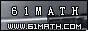Today is . WELCOME: huohai3983/44663  Home | Reg Login | All user | setHomePage | BookmarkHome | Math Test Online | Addition | Subtraction | Multiplication | Division | Time | Worksheet(JPG/PDF) | CAI | Flash Games |Recommendation articleArticle searchHome--maths--Fourth Grade--Improving an Estimate of a sum by rounding

Improving an Estimate of a sum by rounding

Improving an Estimate of a sum by rounding

A quick way to estimate the sum of two numbers is to round each number and then add the rounded numbers. This probably won't be the exact answer but it may be close enough for some purposes.

How to Estimate a sum by rounding.

• Round each term that will be added

An estimate can sometimes be improved. If the sum of 345 + 440 were estimated, we would round 345 to 300 and 440 to 400. The estimate would be 300 + 400 or 700. Both numbers were rounded down. The number 345 was rounded down by 45 and 440 was rounded down by 40. Adding 45 + 40 gives 85, which rounds to 100. Therefore, a better estimate would be 800. The actual sum is 785.

How to Improve the Estimate.

• Round each term that will be added
• If both are rounded down or both rounded up see if the amount of rounding is more than 50. If it is, add or subtract 100 to the estimate.
• If one number is rounded down and the other is rounded up a closer estimate will not be produced by this method.

Some uses of rounding are:

• Checking to see if you have enough money to buy what you want.
• Getting a rough idea of the correct answer to a problem

#### Estimate the Sum to the nearest thousand.

 +

2006-11-19 12:33:33
Viewed 2633 times CloseGreatmathsites